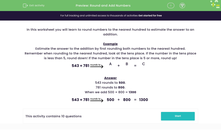### Comprehensive & curriculum aligned

In this worksheet, students learn to round numbers to the nearest hundred in order to estimate the answer to an addition.Key stage:  KS 2

Curriculum topic:   Number: Number and Place Value

Curriculum subtopic:   Round Numbers Up/Down (to 10, 100 or 1000)

Popular topics:   Rounding worksheets

Difficulty level:#### Worksheet Overview

In this worksheet you will learn to round numbers to the nearest hundred to estimate the answer to an addition.

Example

Estimate the answer to the addition by first rounding both numbers to the nearest hundred.

Remember when rounding to the nearest hundred, look at the tens place. If the number in the tens place is less than 5, round down! If the number in the tens place is 5 or more, round up!

543 + 781A

+

 B

=

 C

543 rounds to 500.

781 rounds to 800.

When we add 500 + 800 = 1300

543 + 781500

+

 800

=

 1300

### What is EdPlace?

We're your National Curriculum aligned online education content provider helping each child succeed in English, maths and science from year 1 to GCSE. With an EdPlace account you’ll be able to track and measure progress, helping each child achieve their best. We build confidence and attainment by personalising each child’s learning at a level that suits them.

Get started